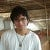# Pattern matching in Golang

Golang syntax was designed to be easy to learn having a few set of keywords, but the reduced syntax generates repeated boilerplate for complex solutions.

Some issues are resolved generating code, but code generators not always are the solution, then we have to be creative to use the language in our advantage, then join me to hack the language.

# Goal

`type Deg { V: float32 } // Degreestype Rad { V: float32 } // RadianssomeDegOrRad := Deg { V: 60.0 }formattedAngle := matchAngle(  someDegOrRad, // only accept Deg or Rad  func (deg) { return deg.FormatString() }  func (rad) { return rad.FormatString() }}`

In other words, the goal is to have pattern matching in Golang with a taste of unions (unfortunately, Golang doesn’t support this feature).

In addition, if we try to provide a angle that is not a Deg or Rad, the syntax analyzer must to show a warning or error message in compile time instead of runtime.

Then, both `Deg` and `Rad` must satisfice an `type Angle interface` , and this must to be closed for more implementations, thus we have a constrained union.

# Why? (What problems do we want to solve?)

`switch v := angle.(type) {case Deg:  // some logic with a Deg structcase Rad:  // some logic with a Rad struct}panic("The angle type is not supported")`

This code pattern looks easy, but if we add an extra type during the evolution of our code, we will need to find every place where the switch is used to add the extra case and the syntax analyzer won’t be able to alert us, i.e. if we forget to add the case somewhere, it will crash in runtime despite it is compiled.

`type Deg { V: float32 } // Sexagesimal Degrees: 0 - 360.0type Rad { V: float32 } // Radians: 0 - 2*pitype Cent { V: float32 } // Centesimal Degrees: Tau: 0 - 400.0someDegOrRad := Deg { V: 60.0 }formattedAngle := matchAngle(  someDegOrRad, // only accept Deg or Rad  func (deg) { return FormatDeg(deg) }  func (rad) { return FormatRad(rad) }  func (cent) { return FormatCent(cent) }}`

If a function needs a new argument, the syntax analyzer will highlight all places we need to update, then we will be confident that it won’t crash in runtime because of a missing case.

# A primitive implementation

`type Angle interface {  // private to avoid new implementation  angle()}type Deg { V: float32 }type Rad { V: float32 }func (d Deg) angle() {}func (r Rad) angle() {}func MatchAngleToString(  angle Matcher[Angle],  deg func(*Deg) String,  rad func(*Rad) String,) R {  switch v := angle.(type) {  case Deg:    return deg(&v)  case Rad:    return rad(&v)  }  panic("MatchAngle: angle type not supported")}`

The `angle()` method is private to make the `Angle` interface closed for more implementations outside the scope. But here there are many scalable issues with this implementation.

## No generic implementation for `MatchAngle`

`func MatchAngle[R any](  angle Angle,  deg func(*Deg) R,  rad func(*Rad) R,) R {  switch v := angle.(type) {  case Deg:    return deg(&v)  case Rad:    return rad(&v)  }  panic("MatchAngle: angle type not supported")}`

## Too much boilerplate for implementing `Angle`

`type Angle interface {  angle() _Angle}type _Angle struct {}func (a _Angle) angle() { return a}type Deg { _Angle, V: float32 }type Rad { _Angle, V: float32 }func MatchAngle[R any](  angle Angle,  deg func(*Deg) R,  rad func(*Rad) R,) R {  switch v := angle.(type) {  case Deg:    return deg(&v)  case Rad:    return rad(&v)  }  panic("MatchAngle: angle type not supported")}`

Adding a new case to the union

Then it is easier to add the `Cent` case:

`type Angle interface {  angle() _Angle}type _Angle struct {}func (a _Angle) angle() { return a}type Deg { _Angle, V: float32 }type Rad { _Angle, V: float32 }type Cent { _Angle, V: float32 }func MatchAngle[R any](  angle Angle,  deg func(*Deg) R,  rad func(*Rad) R,  cent func(*cent) R,) R {  switch v := angle.(type) {  case Deg:    return deg(&v)  case Rad:    return rad(&v)  case Cent:    return cent(&v)  }  panic("MatchAngle: angle type not supported")}`

# Further reducing of the boilerplate

`// private for avoiding new implementationstype _Angle struct {}type Angle Matcher[_Angle]// or with more methods// type Angle interface {//   Matcher[_Angle]//   ToDeg()//   ToRad()//   ToCent()// }type Deg ValuedMatchable[_Angle, float32]type Rad ValuedMatchable[_Angle, float32]type Cent ValuedMatchable[_Angle, float32]func MatchAngle[R any](  angle Matcher[Angle],  deg func(*Deg) R,  rad func(*Rad) R,  cent func(*cent) R,) R {  switch v := angle.(type) {  case Deg:    return deg(&v)  case Rad:    return rad(&v)  case Cent:    return cent(&v)  }  panic("MatchAngle: angle type not supported")}`

The question is “how must `ValuedMatchable` and `Matcher` be implemented?”.

`// Struct base for implementing compositiontype matchable[M ~struct{}] struct{}type Matcher[M ~struct{}] interface {  matcher() matchable[M]}// implementation of Matcher for matchable[M]func (m matchable[M]) matcher() matchable[M] {  return m}// Public Matchable without value.// Allows to compose or subtyping without losing the// Matcher implementation//// example: type NoneAngle Matchable[_Angle]type Matchable[M ~struct{}] struct{ matchable[M] }// An implementation with a valuetype ValuedMatchable[M ~struct{}, V any] struct {  matchable[M]  Val V}`

Conclusions

The manual implementation of `MatchAngle` can not be avoided, it’s implemented once with the benefit of compile safe union handling.

Generics in Golang are not so powerful as in other languages, but we can find tricks for making syntax tools for maintainable code.